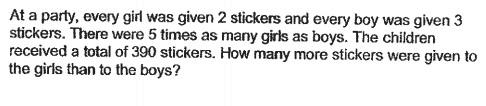QuestionThis as well? can anyone help? thank you

Hi KateM

This is a grouping concept question.

The ratio of girls to boys here is 5 : 1.

What this means, is that for every 5 girls that exist in that party, there will definitely be 1 available boy to be grouped up with these 5 girls. This is what ratios mean and represent. The proportion of things.

So the smallest possible group that can exist with equal number of boys and girls per group would be as such, in that ratio. Since each girl received 2 stickers and boy received 3 stickers, we can see that the total number of stickers in each group of  5 girls and 1 boy would be 13.

We’ve learnt that there were a total of 390 stickers distributed. That would mean that 30 such groups exist. Because 390 divided by 13 is 30.

Hence, the number of stickers given to the girls would be 30 x 5 x 2 = 300

And, the number of stickers given to the girls would be 30 x 1 x 3 = 90

The girls have received 300 – 90 = 210 more stickers than the boys!

Hope this helps!

We provide coaching for small, focus groups of students at Future Achievers Hub at Tampines Junction.

Do check out:

www.futureachievers.com.sg

or call us at +65 83325521,